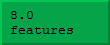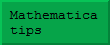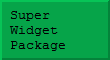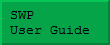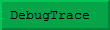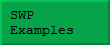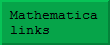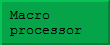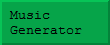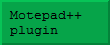Download notebook Consider a StandardForm expression such as:It is very easy to translate this cell into TraditionalForm to obtain: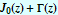Traditional form is much more pleasing to the eye, but it can be hard to input or edit expressions in Traditional form because these cells contain additional information to remove the ambiguity inherent in ordinary mathematics. Consider the following example: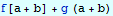if we ask Mathematica to translate this into TraditionalForm, we get: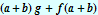Because TraditionalForm follows conventional mathematical notation and uses round parentheses for grouping expressions and for function application, there is an inherent ambiguity in such expressions. This is amplified by the fact that Mathematica is used in such a wide range of applications – the conventional notation of one discipline can overlap with the entirely different notation of another. To avoid this, it is probably best to forget TraditionalForm, except as a way to polish results for transfer to a final report. However, it is possible to use a great deal of traditional mathematical notation in StandardForm – it is simply necessary to define what it means. For example: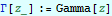This definition will allow you to input gamma functions using the traditional notation. They will be converted as soon as the expression reaches the kernel. Of course, this will not help if you are expecting results from Mathematica involving Gamma functions. There are several ways of dealing with this situation. One way is to program the frontend to display gamma functions in your notation (normal textbook notation!). An alternative, simpler idea, is not to use the above definition, so that an expression such as: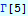is not interpreted by Mathematica at all. This is another example of working with an undefined function. Notice that without a definition, Mathematica will not 'know' that this can be evaluated to 24, and this can be useful in its own right – Mathematica's relentless evaluation can get in the way sometimes!. We now equip our 'toolbox' (see above) with the following sets of transformation rules: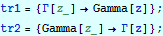Notice that both lists of transformations will typically contain several items to cover all the bits of mathematical notation that you want to translate. Now we can use our private notation and use Mathematica to simplify it: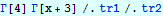The important idea to learn here is that the clumsy spelled-out names of Mathematica are not an impediment to working in neater ways – they simply avoid gobbling notation, such as the Greek letters, for one concept, thereby making it hard to use a symbol for some other notation. All those traditional math symbols, Greek and Gothic script, etc. are there for a reason – use them!